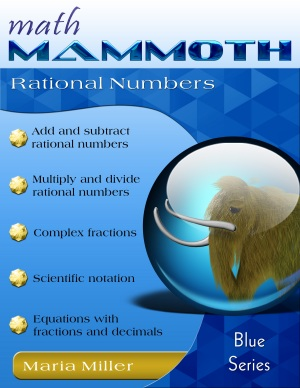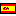^
You are here: HomeBlue Series → Rational Numbers

# Math Mammoth Rational Numbers76 pages

## Prices & ordering

Printed copy \$10.80

(credit cards, Google Pay, Paypal, PO)Este libro en español

In Math Mammoth Rational Numbers we study rational numbers, which are numbers that can be written as a ratio of two integers. All fractions and whole numbers are rational numbers, and so are percentages and decimals (except non-ending non-repeating decimals). Hopefully, students already know a lot about rational numbers and how to calculate with them. Our focus in this book is to extend that knowledge to negative fractions and negative decimals.

The first lesson, Fractions and Decimals, reviews methods of converting fractions to decimals and decimals to fractions.

Next, we present the definition of a rational number, how to convert rational numbers back and forth between their fractional and decimal forms, and a bit about repeating decimals (most fractions become repeating decimals when written as decimals). The next lesson deals with adding and subtracting rational numbers, with an emphasis on adding and subtracting negative fractions and decimals.

The next two lessons are about multiplying and dividing rational numbers. The first of the two focuses on basic multiplication and division with negative fractions and decimals. The second of the two compares multiplying and dividing in decimal notation to multiplying and dividing in fraction notation. Students come to realize that, though the calculations – and even the answers – may look very different, the answers are equal. The lesson also presents problems that mix decimals, fractions, and percentages, and deals with real-life contexts for the problems and the importance of pre-estimating what a reasonable answer would be.

The lesson Multiple Operations with Rational Numbers reviews the order of operations and applies it to fraction and decimal problems with more than one operation. It also presents a simple method to solve complex fractions, which are fractions that contain another fraction, either in the numerator, in the denominator, or in both.

After a lesson on scientific notation, the instructional portion of the book concludes with two lessons on solving simple equations that involve fractions and decimals.

I have made a set of videos to match the lessons of this book. You can access the videos here (free).

## Better Yet - Bundle Deals!

*BONUS*: Buy any bundle listed above, and get the fully upgraded Soft-Pak software for FREE (includes 4 math and 2 language arts programs, complete with on screen and printable options).

## How and where to order

You can buy Math Mammoth books at:

• Here at MathMammoth.com website — simply use the "Add to cart" buttons you see on the product pages.
• Rainbow Resource carries printed copies for the Light Blue series books, plus several CDs (Light Blue and Blue series).
• At Teachers Pay Teachers you can purchase the Light Blue Series downloads, plus topical units.
• Lulu sells printed copies for most of the Math Mammoth materials (various series).

By purchasing any of the books, permission IS granted for the teacher (or parent) to reproduce this material to be used with his/her students in a teaching situation; not for commercial resale. However, you are not permitted to share the material with another teacher.

In other words, you are permitted to make copies for the students/children you are teaching, but not for other teachers' usage.

Math Mammoth books are PDF files. You will need Adobe Reader to view them, including if you use a Mac or Linux. You can try other PDF viewers, but they seem to either omit or mess up some of the images.
WAIT!

Receive my monthly collection of math tips & resources directly in your inbox — and get a FREE Math Mammoth book!You can unsubscribe at any time.

### Math Mammoth Tour

Confused about the different options? Take a virtual email tour around Math Mammoth! You'll receive:

An initial email to download your GIFT of over 400 free worksheets and sample pages from my books. Six other "TOURSTOP" emails that explain the important things and commonly asked questions concerning Math Mammoth curriculum. (Find out the differences between all these different-colored series!)

This way, you'll have time to digest the information over one or two weeks, plus an opportunity to ask me personally about the curriculum.
A monthly collection of math teaching tips & Math Mammoth updates (unsubscribe any time)### "Mini" Math Teaching Course

This is a little "virtual" 2-week course, where you will receive emails on important topics on teaching math, including:

- How to help a student who is behind
- Troubles with word problems
- Teaching multiplication tables
- Why fractions are so difficult
- The value of mistakes
- Should you use timed tests
- And more!

A GIFT of over 400 free worksheets and sample pages from my books right in the very beginning.A monthly collection of math teaching tips & Math Mammoth updates (unsubscribe any time)
Enter your email to receive math teaching tips, resources, Math Mammoth news & sales, humor, and more! I tend to send out these tips about once monthly, near the beginning of the month, but occasionally you may hear from me twice per month (and sometimes less often).• A GIFT of over 400 free worksheets and sample pages from my books.